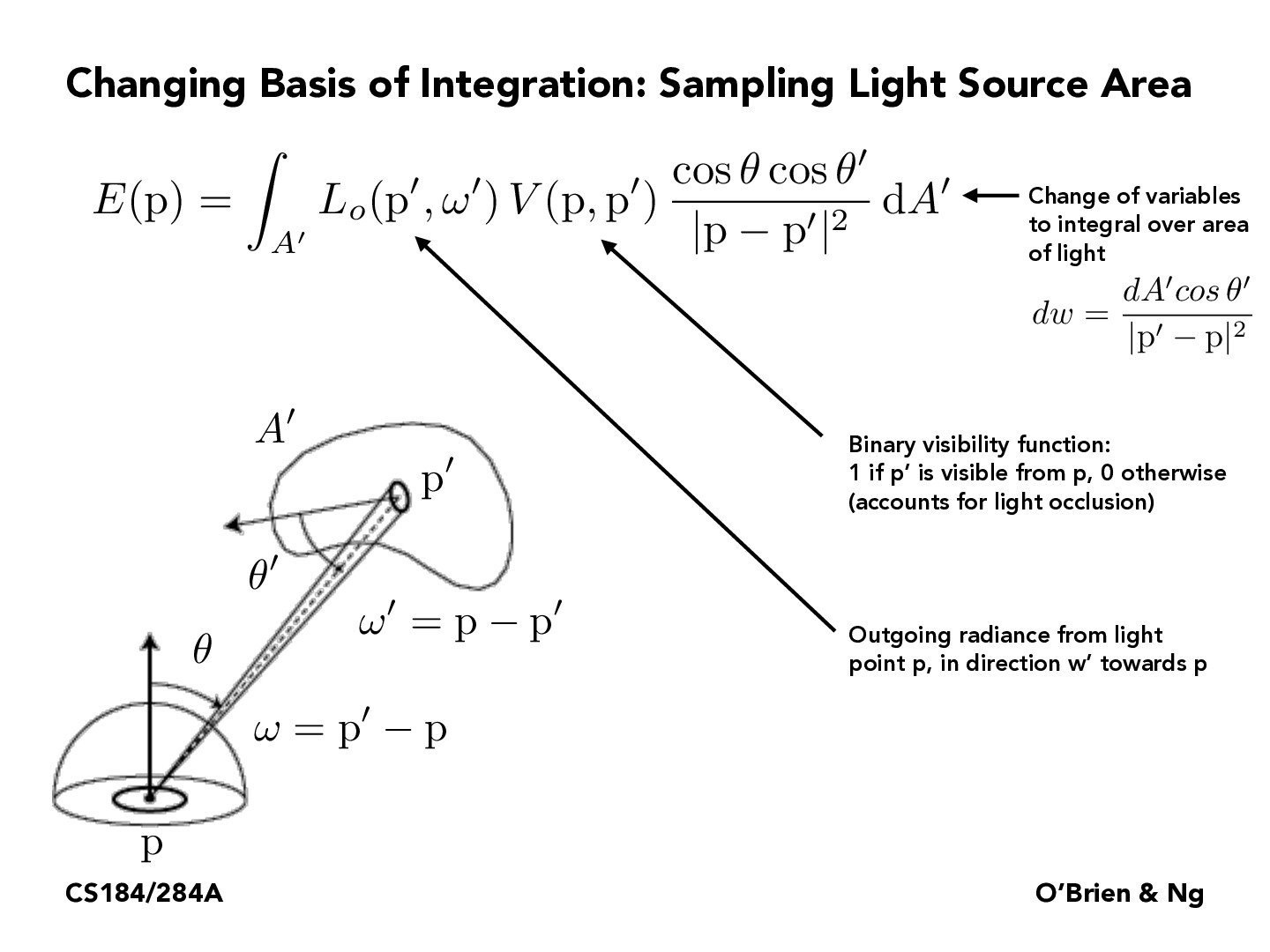Lecture 12: Monte Carlo Integration (45)rsha256

To what level of understanding should we have on this level of math?

What is $A'$ and how would we calculate it?curiousdragon

I think $A^{\prime}$ here represents the light source area, which we're integrating over. I assume in terms of calculating it, this will depend on what we know about what the light source looks like.

On that note, what happens if the light source is actually a point source (e.g. we're modeling it as a point source because it might be very far away but still very powerful)? Then the area of the light source is zero, but it should still have some contribution to the scene. However, under our approach, this might give us a weird (infinity?) answer. Do we treat this as a special case?SeanW0823

Is the caption for function L(p',w') supposed to be "Outgoing radiance from light point p'" instead of "Outgoing radiance from light point p"?

You must be enrolled in the course to comment